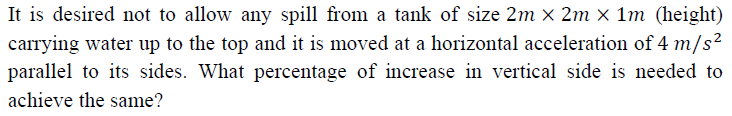51. Study of impact of jets and of velocity triangles is based on Newton’s law which states: Force = mass X acceleration. For practical applications, the following features are emphasized.

1. Change of velocity takes place in a finite time duration
2. Rather than the ‘mass’, the ratio of ‘weight’ and ‘g’ is used.
3. Change of velocity normal to the direction of the impacting inflow is also relevant to the computations

Which of the above are correct?
(a) 1 and 2 only
(b) 1 and 3 only
(c) 2 and 3 only
(d) 1, 2 and 3

52. Consider the following statements:
1. The deformation of structures subjected to several simultaneously applied loads may be obtained by algebraically summing the deformations due to the individual loads.
2. Such superposition holds both for linear and nonlinear systems.

Which of the above statements is/are correct?
(a) 1 only
(b) 2 only
(c) Both 1 and 2
(d) Neither 1 nor 2

53. Water is supplied from a height of 5.2 m at the rate of 100 lps to a hydraulic ram
that delivers 7 lps to a height of 24 m above the ram. The head loss at the supply
pipe is 0.2 m and the delivery pipe has a loss of 1.0 m. The efficiency of the ram is
(a) 22.8 %
(b) 29.8 %
(c) 35.0 %
(d) 39.6 %

54.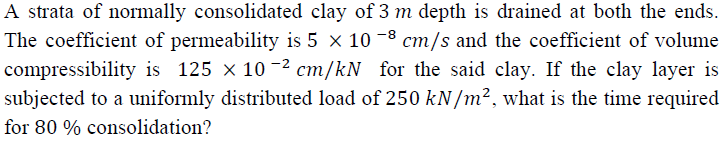(a) 55.2 days
(b) 36.9 days
(c) 156.0 days
(d) 220.8 days

55. A turbine develops 500 kW power under a net head of 30 m . If the overall efficiency of the turbine is 0.83, the discharge through the turbine is nearly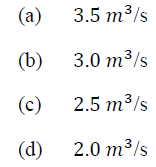56.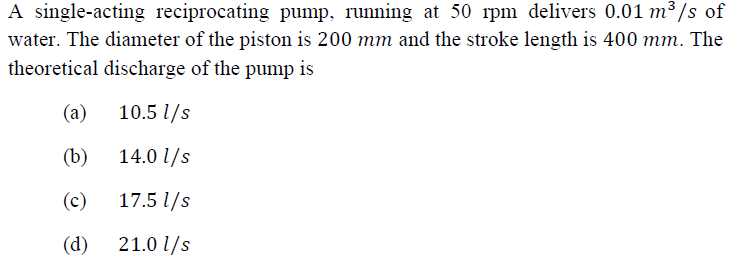57. The Hardy Cross method of analysis of pipe net works requires that:
1. Flow into any junction equals the flow out of it
2. Flow in each pipe has head loss according to respective flow equations
3. Momentum principle is followed in each loop
4. Algebraic sum of the head losses around any closed loop is zero

Which of the above statements are correct?
(a) 1, 2 and 3
(b) 1, 3 and 4
(c) 1, 2 and 4
(d) 2, 3 and 4

58.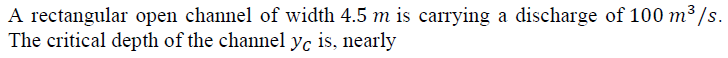(a) 3.4 m
(b) 3.7 m
(c) 4.0 m
(d) 4.2 m

59.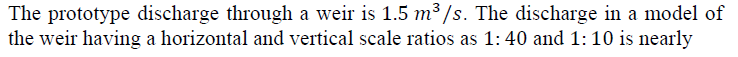(a) 1.5 lps
(b) 1.2 lps
(c) 1.0 lps
(d) 0.9 lps